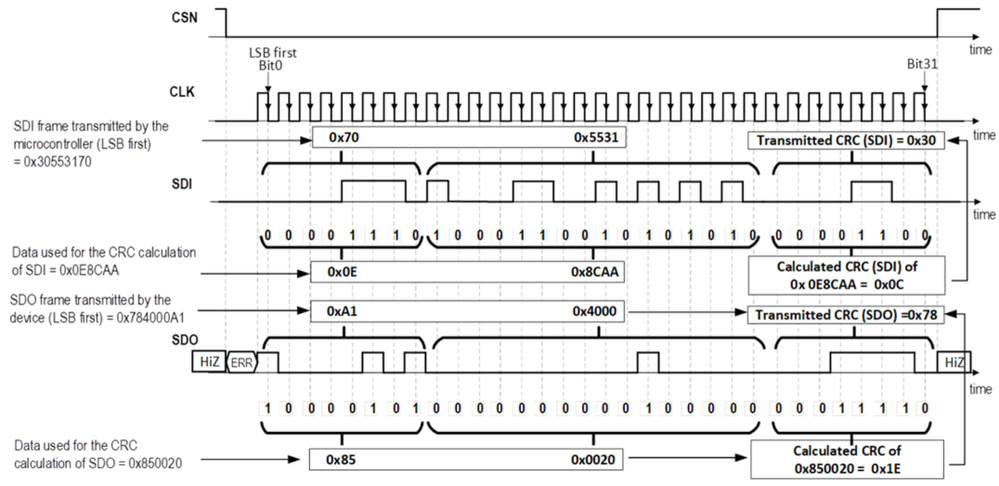# DC motor system IC TLE9560/1/2- Can you give an example of CRC calculation?ModeratorModerator# DC motor system IC TLE9560/1/2- Can you give an example of CRC calculation?

The TLE956x family implements a CRC8 wit the polynomial 2Fh.

In this example, the microcontroller reads the status register family and product identifier (FAM_PROD_STAT) of the TLE9562-3QX, while the cyclic redundancy check (CRC) is enabled.

It shows how the device and the microcontroller calculate the CRC.

Microcontroller/device CRC calculation for SDI:

1. Address byte D0 ... D7:
1. The offset address of the FAM_PROD_STAT register is 0b111 0000
2. The bit 7 is 0 for a read command
3. Therefore the address byte is: 0b0111 0000 = 0x70
• For a read command the payload can be arbitrarily chosen by the microcontroller. In this example, the payload is equal to 0x5531.
3. CRC calculation:
1. The CRC takes into account the BIT0 to BIT23 in this order, as if the frame was sent with the most significant (MSB) bit first. Therefore the CRC data is 0x0E8CAA.
2. The CRC result is 0x0C
3. 0x0C corresponds to 0x30 with the least significant bit (LSB) first

Therefore the microcontroller must send the SPI frame (SDI) 0x30553170 for a correct CRC.

Microcontroller/device CRC calculation for SDO:

1. In this example of the TLE9562-3QX transmits on SDO:
1. The status information field = 0xA1